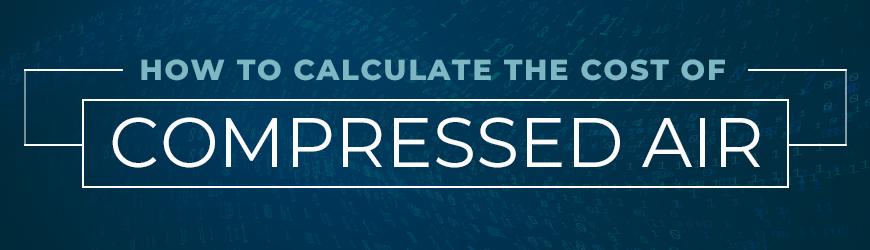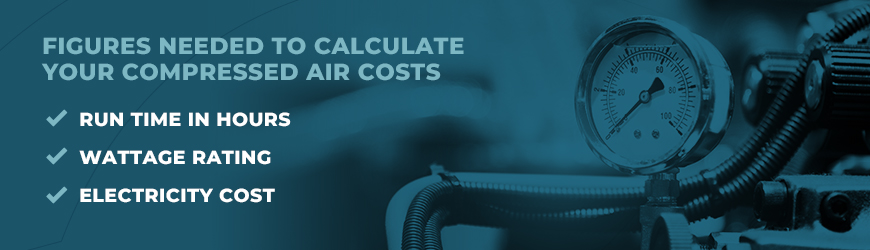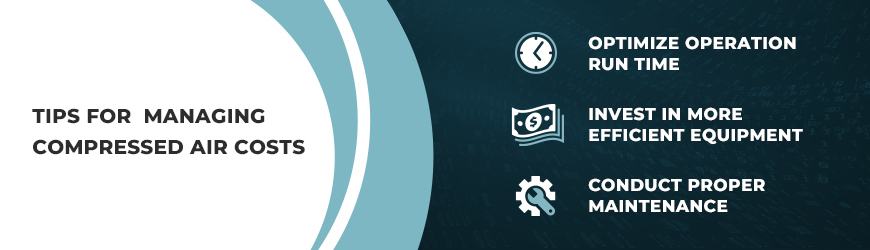+607-555 0515sales@kaishan.com.my

## How To Calculate The Cost Of Compressed AirChoosing the right air compressor is a decision that can have a significant long-term impact on your energy costs. Why? Compressed air generation accounts for as much as 30% of a facility’s annual electric bill.

However, the air compressor cost is a relatively small percentage of that equipment’s lifetime cost. In general, about 75% of an air compressor’s lifetime cost will go towards powering it, with the remainder typically split between the upfront equipment cost and maintenance and repairs.

You can manage the cost of generating compressed air with several best practices. The first step is knowing how to calculate cost of compressed air, and using that information to set benchmarks and reduce energy expenditures.

Calculating the Cost of Compressed Air

In most manufacturing facilities, compressed air is as necessary for most processes and operations as electricity and water. Compressed air is used for cleaning, to power pneumatic tools and processes, in cleanrooms, for cooling and more. Without compressed air, a manufacturing facility would be rendered virtually inoperable. When you consider this reality, the need to efficiently manage the costs of generating that air makes more sense.

Let’s first look at how to calculate compressed air cost, and then we’ll share some tips for reducing those costs.You’ll need a few figures and specifications in order to make your calculations. These are:
• Run time in hours — Once you have determined the run time in hours, you can use this information to extrapolate costs per week, month and year.
• Wattage rating — This number should be stamped on the compressor or included in the accompanying documentation.
• Electricity cost — This will usually be the cost per kilowatt hour, which generally runs between 7 and 25 cents depending on your location and utility provider.
With these figures in hand, carry out the following operations:
1. Multiply wattage by current to determine the total power consumed in one hour.
2. Multiply the above number by the number of operational hours in the time period for which you want to determine costs.
3. The above number will be in watt hours. Divide that number by 1,000 to arrive at the number of kilowatt hours.
4. Multiply the kilowatt hours used by the cost of electricity. This number will be the cost of operating your compressor over a given time period.
As you’ll see, multiplying this cost over the lifespan of a compressor — which can be as much as 20 years — accounts for a significant expenditure.

Tips for Managing Compressed Air Costs